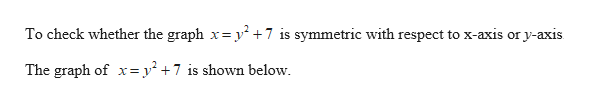# For Exercise, determine whether the graph of the equation is symmetric with respect to the x-axis or y-axis.x = y2 + 7

Question
1 views

For Exercise, determine whether the graph of the equation is symmetric with respect to the x-axis or y-axis.

x = y2 + 7

check_circle

Step 1help_outlineImage TranscriptioncloseTo check whether the graph x= y +7 is symmetric with respect to x-axis or y-axis The graph of x= y² +7 is shown below. fullscreen
Step 2

### Want to see the full answer?

See Solution

#### Want to see this answer and more?

Solutions are written by subject experts who are available 24/7. Questions are typically answered within 1 hour.*

See Solution
*Response times may vary by subject and question.
Tagged in

### Other